# Estimating decimals#### All in One Place

Everything you need for better grades in university, high school and elementary.#### Learn with Ease

Made in Canada with help for all provincial curriculums, so you can study in confidence.#### Instant and Unlimited Help

0/2
##### Intros
###### Lessons
1. Introduction to Estimating Decimals:
2. How to estimate decimal values using number lines
3. How to round decimal numbers
0/15
##### Examples
###### Lessons
1. Using number lines to round decimals
Use the number line to help you round the decimal. Draw an arrow pointing to:
1. the nearest whole number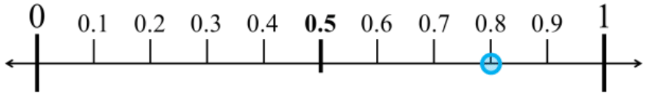2. the nearest tenth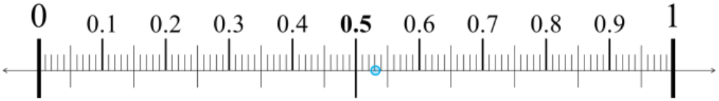3. the nearest whole number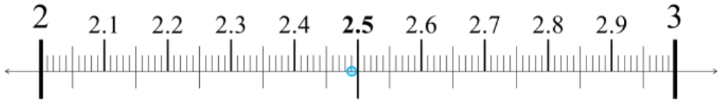4. the nearest tenth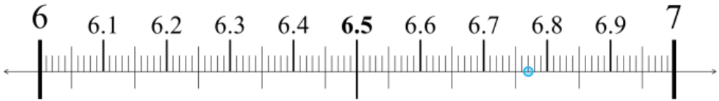2. Rounding decimals to different place values
Round the decimal to the specified place value.
1. Round 51.4 to the nearest whole number.
2. Round 36.78 to the nearest tenth
3. Round 6.29 to the nearest whole number.
4. Round 2.95 to the nearest tenth.
3. Comparing relative decimal values
Compare the relative decimal and fraction values to answer.
1. Write whether each decimal is more than, less than, or equal to $\large \frac{1}{2}$
1. 0.49
2. 0.50
3. 0.68
2. Determine if these decimals are more than, less than, or equal to $\large \frac{1}{4}$
1. 0.4
2. 0.16
3. 0.25
3. A strawberry jam recipe needs 2$\large \frac{3}{4}$ pounds of strawberries. Margot weighs three different boxes at the store. Which box should she buy so that she has enough for the recipe? Why?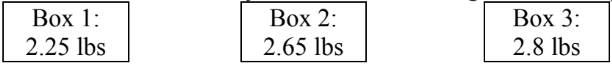4. Estimating decimal values from picture representations
Estimate the decimal value represented by the picture. Answer using the $\approx$symbol.
Hint: Try to create equal divisions.
1.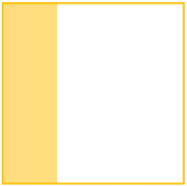2.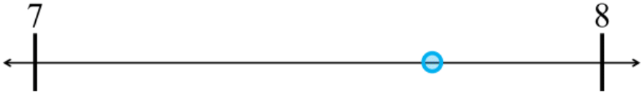3.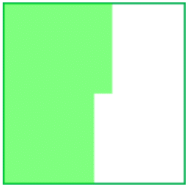4.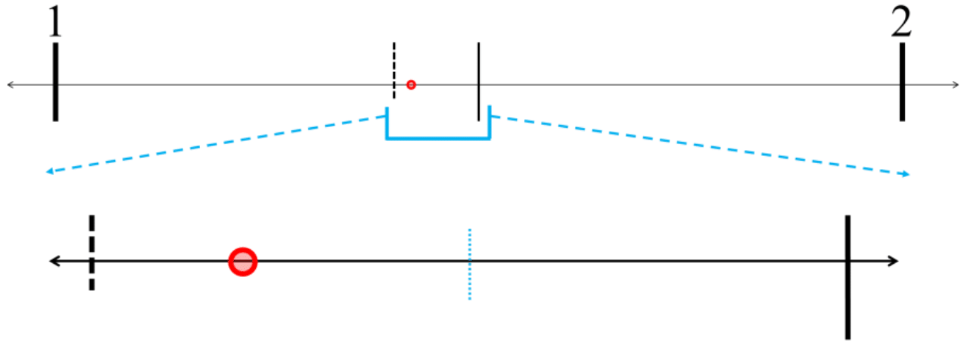0%
##### Practice
###### Topic Notes

In this lesson, we will learn:

• How to use number lines to help estimate decimal values (to the closest whole number or closest tenth)
• How to round decimal numbers to the nearest whole number, or the nearest tenth

Notes:

• To estimate means to roughly guess the value (or a rough calculation)
• For estimating decimals, have less decimal place values in your number
• Rounding is one strategy for estimation; by making numbers less exact (less precise), they become simpler or easier to do math with
• We use the symbol $\approx$ meaning "about equal to" for estimation and rounding
• Ex. 39 is about equal to the even number 40; 39 $\approx$ 40

• We can use number lines to help estimate decimal values
• Find the closest distance to the nearest whole number OR the nearest tenth
• Ex. rounding 0.3 to the nearest whole number, is it closer to 0 or 1?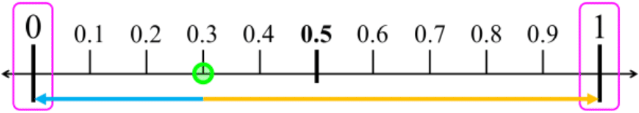• 0.3 is closer to 0 than it is to 1. Therefore, 0.3 $\approx$ 0
• Ex. rounding 0.66 to the nearest tenth, is it closer to 0.6 or 0.7?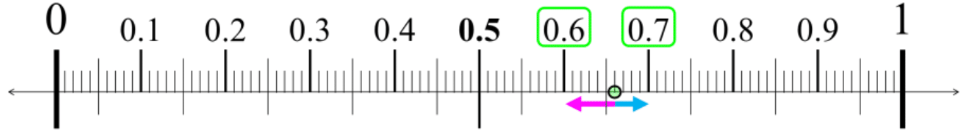• 0.66 is closer to 7 than it is to 6. Therefore, 0.66 $\approx$ 0.7
• The steps for rounding numbers:
1. Look at the place value you are rounding to ("target").
$\quad \, \longrightarrow \;$ start writing a new rounded number, where:
$\quad \, \longrightarrow \;$ any smaller place values (to the right) can be changed to zero
$\quad \, \longrightarrow \;$ any bigger place values (to the left) can be kept
2. Look at the place value to the right of where you are rounding to.
1. if the digit is $\geq 5$, round up (increase the target by 1)
2. if the digit is < 5, round down (keep the target the same)
3. Any trailing zeroes in decimals can be removed (i.e. 0.50 = 0.5; 2.0 = 2)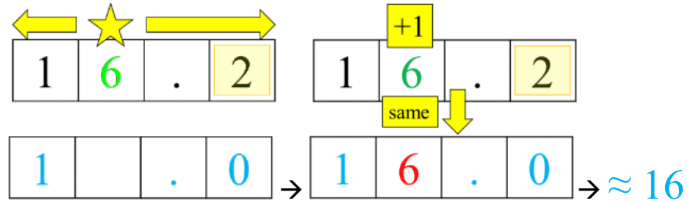• When your target digit is 9, if you round up then you will regroup to the next place value up.
• Ex. Rounding 0.97 to nearest tenth $\, \longrightarrow \,$ round up from 0.9 to 1.0; 0.97 $\approx$ 1.0